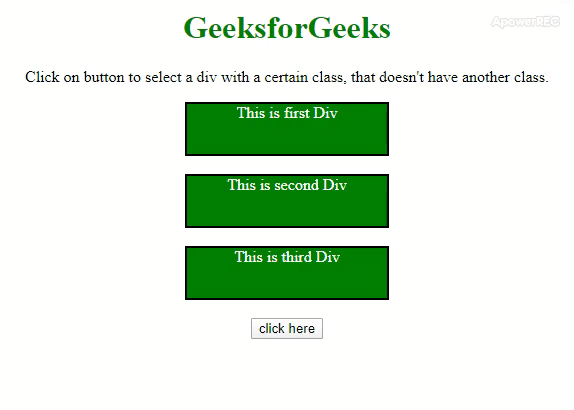# How to select a div with a certain class using jQuery ?

• Last Updated : 27 Oct, 2021

Given a HTML document containing many div element with classes. Here the task is to select a div with a certain class, that doesn’t have another class. There are two approaches to solve this problem. In one of them, we will use a method and in the other one, we will use not selector.

Approach 1: First, select the DIV with certain class using jQuery Selector and then use :not selector to ignore the elements of specific class.

• Example: This example implements the above approach.

## html

 ```<``html``>` `<``head``>``    ``<``title``>``        ``Select a div with a certain class,``        ``that doesn't have another class.``    ````    ``<``style``>``        ``body {``            ``text-align: center;``        ``}``        ` `        ``h1 {``            ``color: green;``        ``}``        ` `        ``.div {``            ``background: green;``            ``height: 50px;``            ``width: 200px;``            ``margin: 0 auto;``            ``color: white;``            ``border: 2px solid black;``        ``}``    ````    ``<``script` `src` `=``"https://ajax.googleapis.com/ajax/libs/jquery/3.4.0/jquery.min.js"``>``    ````` `<``body``>``    ``<``h1``>``        ``GeeksforGeeks``    ````    `  `<``p``>``          ``Click on button to select a div with a certain``        ``class, that doesn't have another class.``    ```  `  ` `    ````    ``<``div` `class``=``"first div"``>``        ``This is first Div``    ````    ``<``br``>``    ``<``div` `class``=``"second div"``>``        ``This is second Div``    ````    ``<``br``>``    ``<``div` `class``=``"third div"``>``        ``This is third Div``    ````    ``<``br``>``    ``<``button` `onClick``=``"GFG_Fun()"``>``        ``click here``    ````    ``<``br``>``    ``<``p` `id``=``"geeks"``>``    ```  `    ``<``script``>``       ` `        ``/* main function */``        ``function GFG_Fun() {``          ` `            ``/* using the :not selector */``            ``\$('.div:not(.first)')``            ``.css("background-color", "#173F5F");``            ``\$('#geeks')``            ``.text("DIV Box of class 'first' is not selected.");``        ``}``    ````` ``
• Output:Approach 2: First, select the DIV with certain class using jQuery Selector and then Use .not() method to ignore the elements of specific class.

• Example: This example implements the above approach.

## html

 ```<``html``>` `<``head``>``    ``<``title``>``        ``Select a div with a certain class,``        ``that doesn't have another class.``    ````    ``<``style``>``        ``body {``            ``text-align: center;``        ``}``        ` `        ``h1 {``            ``color: green;``        ``}``        ` `        ``.div {``            ``background: green;``            ``height: 50px;``            ``width: 200px;``            ``margin: 0 auto;``            ``color: white;``            ``border: 2px solid black;``        ``}``    ````    ``<``script` `src` `=``"https://ajax.googleapis.com/ajax/libs/jquery/3.4.0/jquery.min.js"``>``    ````` `<``body``>``    ``<``h1``>``        ``GeeksforGeeks``    ````    `  `<``p``>``        ``Click on button to select a div with a certain``        ``class, that doesn't have another class.``    ```   `    ````    ``<``div` `class``=``"first div"``>``        ``This is first Div``    ````    ``<``br``>``    ``<``div` `class``=``"second div"``>``        ``This is second Div``    ````    ``<``br``>``    ``<``div` `class``=``"third div"``>``        ``This is third Div``    ````    ``<``br``>``    ``<``button` `onClick``=``"GFG_Fun()"``>``        ``click here``    ````    ``<``br``>``    ``<``p` `id``=``"geeks"``>``    ```  `    ``<``script``>``        ` `        ``/* main function */``        ``function GFG_Fun() {``            ` `            ``/* using the .not() method */``            ``\$('.div').not('.first')``            ``.css("background-color", "#173F5F");``            ``\$('#GFG_DOWN')``            ``.text("DIV Box of class 'first' is not selected.");``        ``}``    ````` `                   `
• Output:My Personal Notes arrow_drop_up